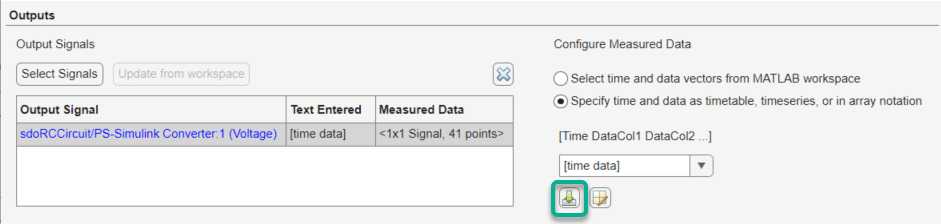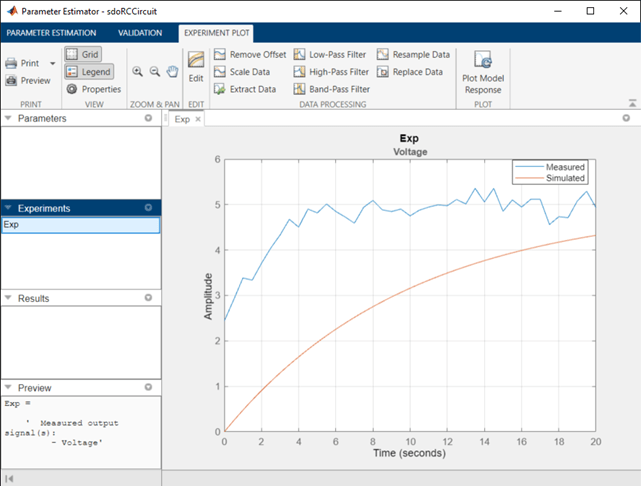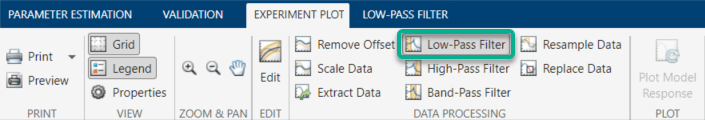# Importing and Preprocessing Experiment Data (GUI)

This example shows how to create an estimation experiment from measured data stored in a file and how to preprocess the measured data. You use the imported data to estimate the parameters of a simple RC circuit.

This example requires Simscape™ software.

### RC Circuit Model

The Simulink® model, `sdoRCCircuit`, models a simple resistor-capacitor (RC) circuit.

```open_system('sdoRCCircuit'); ```You use measured data to estimate the RC model parameter and state values.

Measured output data:

• Capacitor voltage, output of the PS-Simulink Converter block

Parameter:

• Capacitance, `C1`, used by the C1 block

State:

• Initial voltage of the capacitor

### Define the Estimation Experiment

In this example, you load the measured data from a saved MATLAB® file. The data is also stored in a comma separated variable (csv) text file. You can also load the measured data directly from text or Excel® files.

First load the measured data from the MATLAB file, the file defines two variables, `time` and `data` that specify the measured capacitor voltage.

```load sdoRCCircuit_ExperimentData ```

To launch the Parameter Estimator, in the Simulink model window, in the Apps gallery, under Control Systems, click Parameter Estimator.Click New Experiment to create an estimation experiment that contains the measured data. A variable `Exp` is created in the Parameter Estimator and a dialog to edit the experiment opens.The experiment editor contains sections to specify measured output data and sections to optionally specify experiment initial states and parameters.

The experiment editor automatically adds measured output signals for model root level ports and logged model signals. Click Select Signals to add additional measured outputs if needed. For this example the capacitor voltage signal is logged in the model and already added to the experiment.

Specify the measured capacitor voltage by typing `[time data]` in the edit field. This uses the MATLAB variables `time` and `data` loaded from file earlier to specify the measured capacitor voltage. Measured data is specified as a matrix where the 1st column is time and subsequent columns signal data.Alternatively, you can specify the measured capacitor voltage variables by loading the measured data directly from text or Excel files. Click the import button to open a file browser and select `sdoRCCircuit_ExperimentData.csv` file.A tool for importing column data from a file opens. The first column selected for import is used to specify the signal time, subsequent columns selected for import are used to specify the signal data. Select the `time` and `data` columns and click Import Selection.In the experiment editor click Plot & Simulate to plot the measured experiment data and the simulated model response.The experiment plot shows that the simulated data does not match the measured data. The plot also shows that the model initial state is not correct and needs to be estimated (the measured and simulated voltages at time 0 are significantly different). From the experiment editor, click Select Initial States to open a dialog to select model initial states; select the `sdoRCCircuit.C1.vc` state and click Ok to add the state to the experiment.### Preprocess the Experiment Data

The measured data contains high frequency noise that you can remove using a low-pass filter. Click the Experiment Plot tab and select Low Pass Filter.This opens the Low-Pass Filter tool. The upper axis shows the signal FFT, the lower axis shows the signals. The original signal is shown in blue and the filtered signal in red. Adjust the filter bandwidth by either typing a value in the Normalized cutoff frequency edit field or clicking and dragging the yellow patch edge. Drag the filter cutoff to 0.4. Click Options and select Zero-phase shift filter to avoid introducing the filter phase shift into the measured data.Click Apply and Close Low-Pass Filter to complete low-pass filtering of the data. The experiment is updated with the filtered signal. You can use other preprocessing tools such as remove offset, scale, and resample to further process the measured data. For this example low-pass filtering is sufficient.

### Estimate Model Parameter Values

With the experiment data configured and preprocessed you can now run an estimation. First select parameters to estimate. Click the Parameter Estimation tab and select Select Parameters. A dialog to specify model parameters for estimation opens. Click Select Parameters and select, `C1`, the circuit capacitor value. Set the capacitor minimum value to 0 and the initial guess to 460e-6.Click Estimate to start the estimation. You can modify estimation options by setting the Cost Function combobox and clicking More Options.

While the estimation is running, the plots update and a dialog showing estimation progress appears. The progress dialog shows the estimation iterations, the number of times the model has been evaluated (F-count), and the estimation cost at each iteration.After a number of iterations the estimation converges and terminates. The model is updated with the estimated parameters and the estimation results are saved in the data browser.

### Related Examples

To learn how to estimate model parameters using the `sdo.optimize` command, see Estimate Model Parameters and Initial States (Code).

Close the model.

```bdclose('sdoRCCircuit') ```